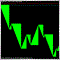# Counting Candles - page 22023

moneycode:

I don't think he needs the time for each bar. He just needs the bar count (renko bar's) to restart at a pre-given time, each day. The bars after the starting bar would not be time related, only 1 bar each day would be time related.

(note* Though renko can also be based on closing prices and a closing price is time related)

Yes this is exactly what i am looking for.2023

WHRoeder:

Does your Renko generator create charts with valid timestamps? Then there's no difference.

As for counting how many bars for the higher time frame, try

```  int    counted_bars=IndicatorCounted();
for(int i = Bars -1 -counted_bars; i>=0;i--){
datetime now = Time[i],
nowTF = now - now % (60 * TimeFrame);
period[i] = iBarShift(NULL,0, nowTF);
}```

I have tried the above code, but it has two problems.

1- the last candle is numeric as 1 and the before last is 2 and so on... Actually i want to count candles so if we count it on normal time frame such as H1 and the last candle is for example on 8 O'clock, the number of candle should be 8 and not 1.

2- This code that you advised me, has not any loop like above pictures. I want to recount candles every day and it should not be related to the past days. For example by your code if on normal H1 chart we have 8 O'clock, it must has the value 8 for current day and for previous day on the same 8 O'clock. but your code gives the value 1 for current candle and 24 for previous day on 8 O'clock.

I hope that you understood what i mean.

Best regards2023

I have found myself a solution for that problem. If anybody need simething like this can use the below code and it works perfect:

If you want to use the below code on Renko please wipeout 1+ on

`       period[i]=1+iBarshift(Null,0,iTime(Symbol(),TimeFrame,y)-iBarshift(Null,0,Time[i]))  ;`

So the first candle after Timeframe period starting will be 1.

But remember if you want to use it on normal charts the number of first candle on H1 timeframe after 00:00 O'Clock will be 0 and the number of bar that opens on 1:00 will be 1.

So if you want that Bar on 00:00 O'Clock has the value 1, you will need 1+ on the code like above.

```//----
#property indicator_separate_window
#property indicator_buffers 1
#property indicator_color1 DodgerBlue
//----
extern int TimeFrame=1440;

//----
double period[];
//+------------------------------------------------------------------+
//| Custom indicator initialization function                         |
//+------------------------------------------------------------------+
int init()
{

//---- 3 additional buffers are used for counting.
//---- indicator lines
SetIndexStyle(0, DRAW_HISTOGRAM);
SetIndexBuffer(0, period);
//---- name for DataWindow and indicator subwindow label
switch(TimeFrame)
{
case 1 : string TimeFrameStr="Period_M1"; break;
case 5 : TimeFrameStr="Period_M5"; break;
case 15 : TimeFrameStr="Period_M15"; break;
case 30 : TimeFrameStr="Period_M30"; break;
case 60 : TimeFrameStr="Period_H1"; break;
case 240 : TimeFrameStr="Period_H4"; break;
case 1440 : TimeFrameStr="Period_D1"; break;
case 10080 : TimeFrameStr="Period_W1"; break;
case 43200 : TimeFrameStr="Period_MN1"; break;
default : TimeFrameStr="Current Timeframe";
}
}
//----
return(0);
//+------------------------------------------------------------------+
//| MTF RSI                                                          |
//+------------------------------------------------------------------+
int start()
{
datetime TimeArray[];
int    i,limit,y=0,counted_bars=IndicatorCounted();
int sum=0;
// Plot defined time frame on to current time frame
ArrayCopySeries(TimeArray,MODE_TIME,Symbol(),TimeFrame);
limit=Bars-counted_bars;
for(i=0,y=0;i<limit;i++)
{
if (Time[i]<TimeArray[y]) y++;

period[i]=1+iBarshift(Null,0,iTime(Symbol(),TimeFrame,y))-iBarshift(Null,0,Time[i])  ;
}
//----
return(0);
}```